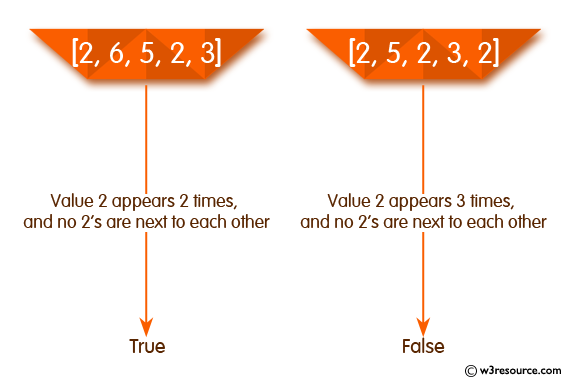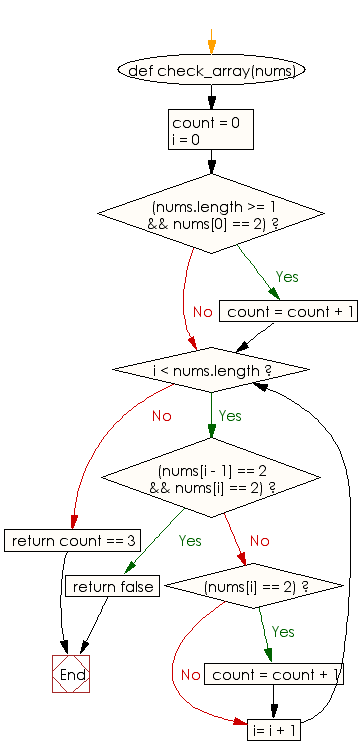﻿ Ruby Array exercises: Check whether the value 2 appears in a given array of integers exactly 2 times, and no 2's are next to each other - w3resource# Ruby Array Exercises: Check whether the value 2 appears in a given array of integers exactly 2 times, and no 2's are next to each other

## Ruby Array: Exercise-41 with Solution

Write a Ruby program to check whether the value 2 appears in a given array of integers exactly 2 times, and no 2's are next to each other.Ruby Code:

``````def check_array(nums)
count = 0
i = 0
if(nums.length >= 1 && nums == 2)
count = count + 1
end
while i < nums.length
if(nums[i - 1] == 2 && nums[i] == 2)
return false
end
if(nums[i] == 2)
count = count + 1
end
i= i + 1
end
return count == 3
end
print check_array([2, 1, 2, 5, 6]),"\n"
print check_array([2, 6, 5, 2, 3]),"\n"
print check_array([2, 5, 2, 3, 2]),"\n"
``````

Output:

```true
true
false
```

Flowchart:Ruby Code Editor: